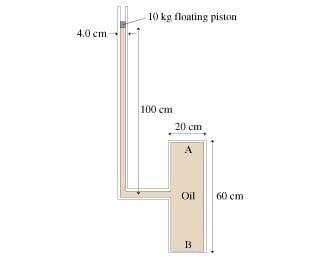# Pressure & Force

## Homework Statement

How much force does the fluid exert on the end of the cylinder at A?## The Attempt at a Solution

p= po + density*g*d
Can I say the pressure is (10kg)(9.80)/pi*(.02m)^2 + (900 kg/m^3)*(9.80)(.70m) and set that equal to F/pi*(.1m)^2 to solve for force? Does the tube enter the container at halfway up?

I cannot see anything wrong with your answer assuming as you have that the tube enters the container at halfway up. Without that assumption its not possible to give a final answer.
Normal atmospheric pressure can be ignored because its effect will be zero on the final force exerted.

I did Pressure= (10kg)*(9.80)/pi*(.02m)^2 + (900kg/m^3)(9.80)(.70m) = 84159.92 N/m^2

84159.92 = F/pi * (.1m)^2 F= 2600 N rounded off with two significant figures because the question tells me to, but this is wrong. What's not quite right?

alphysicist
Homework Helper
Hi bcjochim07,

I think you need to account for the atmospheric pressure that acts above the piston.

ok, I didn't understand why apelling told me I didn't

so Pressure = (10kg)(9.80)/pi*(.02m)^2 + 101300N/m^2 + (900kg/m^3)(9.80)(.70) = 185460

185460 = F/pi* (.1m)^2 F= 5800 N ?

also the second part of the question asks for force at b, so I would do the same sort of thing only with a depth of 1.3m?

alphysicist
Homework Helper
As long as it's safe to assume that these pipes and things are circular in cross section (and that your original assumption about the halfway mark is correct), I think that's right.

Ok so here's what I did for the force at b:

(10kg)(9.80)/pi*(.02m)^2 + 101300 + (1.3m)(9.80)(900 kg/m^3) = P

P= 190752 = F/ pi * (.1m)^2 F= 5992.65 N. This is mastering physics homework, and it says to round it to two significant figures, anybody know how I would do that on a computer?

Oh ok.... scientific notation of course. Does my answer for part b seem logical?

The reason why I suggested ignoring atmospheric pressure is because I was thinking in terms of the resultant force on the cylinder end, whereas you required the force from the liquid only. Sorry to have mislead you.

Does my answer for part b seem ok?

Any thoughts anybody?

alphysicist
Homework Helper
If you're having doubts about it, you can use the pressures at A and B to check that they are consistent, using the same equation:

$$P_B = P_A +\rho g h$$

where h is the depth of B relative to A.

Or you can compare the forces at A and B. The force at B is the force at A (that is the force the top of the container is pusing down on the fluid) plus the weight of the fluid above point B:

\begin{align} F_B &= F_A + m g \nonumber\\ &= F_A + \rho g (\pi r^2) h\nonumber \end{align}

because $\pi r^2 h$ is the volume of the liquid above point B. (Be sure to use the full number for $F_A$, though, not the amount after you rounded off to two digits.)